# Acceleration facts for kids

Kids Encyclopedia Facts
(Redirected from Accelerate)

Acceleration is a measure of how fast velocity changes. Acceleration is the change of velocity divided by the change of time. Acceleration is a vector, and therefore includes both a size and a direction.

## Examples

• An object was moving north at 10 meters per second. The object speeds up and now is moving north at 15 meters per second. The object has accelerated.
• An apple is falling down. It starts falling at 0 meters per second. At the end of the first second, the apple is moving at 9.8 meters per second. The apple has accelerated. At the end of the second second, the apple is moving down at 19.6 meters per second. The apple has accelerated again.
• Jane is walking east at 3 kilometers per hour. Jane's velocity does not change. Jane's acceleration is zero.
• Tom was walking east at 3 kilometers per hour. Tom turns and walks south at 3 kilometers per hour. Tom has had a nonzero acceleration.
• Sally was walking east at 3 kilometers per hour. Sally slows down. After, Sally walks east at 1.5 kilometers per hour. Sally has had a nonzero acceleration.
• Acceleration due to gravity

## Finding acceleration

Acceleration is the rate of change of the velocity of an object. Acceleration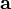$\mathbf{{a}}$ can be found by using: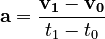$\mathbf{{a}} = {\mathbf{v_1} - \mathbf{v_0} \over { t_1 - t_0 }}$

where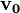$\mathbf{v_0}$ is the velocity at the start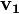$\mathbf{v_1}$ is the velocity at the end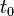$t_0$ is the time at the start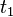$t_1$ is the time at the end

Sometimes the change in velocity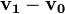$\mathbf{v_1} - \mathbf{v_0}$ is written as Δ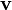$\mathbf{v}$. Sometimes the change in time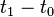${ t_1 - t_0 }$ is written as Δt.

In difficult situations, the acceleration can be calculated using mathematics: in calculus, acceleration is the derivative of the velocity (with respect to time),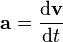$\mathbf{a} = \frac{\mathrm{d}\mathbf{v}}{\mathrm{d}t}$.

### Units of measurement

Acceleration has its own units of measurement. For example, if velocity is measured in meters per second, and if time is measured in seconds, then acceleration is measured in meters per second squared (m/s2).

### Other words

Acceleration can be positive or negative. When the acceleration is negative (but the velocity does not change direction), it is sometimes called deceleration. For example, when a car brakes it decelerates. Physicists usually only use the word "acceleration".

## Newton's second law of motion

Newton's laws of motion are rules for how things move. These rules are called "laws of motion". Isaac Newton is the scientist who first wrote down the main laws of motion. According to Newton's Second Law of Motion, the force something needs to accelerate an object depends on the object's mass (the amount of "stuff" the object is made from or how "heavy" it is). The formula of Newton's Second Law of Motion is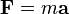$\mathbf{F} = m \mathbf{a}$, where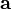$\mathbf{a}$ is the acceleration,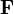$\mathbf{F}$ is the force, and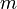$m$ the mass. This formula is very well-known, and it is very important in physics. Newton's Second Law of Motion, in short "Newton's Second Law", is often one of the first things that physics students learn.

## Deceleration

Deceleration is the opposite of acceleration. This means that something slows down instead of speeding up. For example, when a car brakes, it is decelerating.

## Images for kidsAcceleration Facts for Kids. Kiddle Encyclopedia.